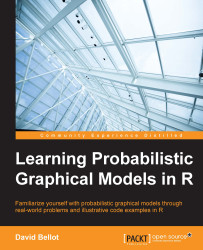•#### Learning Probabilistic Graphical Models in R#### Overview of this book

Probabilistic graphical models (PGM, also known as graphical models) are a marriage between probability theory and graph theory. Generally, PGMs use a graph-based representation. Two branches of graphical representations of distributions are commonly used, namely Bayesian networks and Markov networks. R has many packages to implement graphical models. We’ll start by showing you how to transform a classical statistical model into a modern PGM and then look at how to do exact inference in graphical models. Proceeding, we’ll introduce you to many modern R packages that will help you to perform inference on the models. We will then run a Bayesian linear regression and you’ll see the advantage of going probabilistic when you want to do prediction. Next, you’ll master using R packages and implementing its techniques. Finally, you’ll be presented with machine learning applications that have a direct impact in many fields. Here, we’ll cover clustering and the discovery of hidden information in big data, as well as two important methods, PCA and ICA, to reduce the size of big problems.
Learning Probabilistic Graphical Models in RCreditswww.PacktPub.comPrefaceFree Chapter
Probabilistic ReasoningExact InferenceLearning ParametersBayesian Modeling – Basic ModelsApproximate InferenceBayesian Modeling – Linear ModelsProbabilistic Mixture ModelsAppendixIndex## Principles of the EM algorithm

Because the latent variables are not observed, the likelihood function of such a model is a marginal distribution where we have to sum out (or integrate out) the hidden variables. Marginalization will create dependencies between the variables and make the problem complex to solve.

The EM algorithm deals with this problem essentially by filling-in missing data with their expected values, given a distribution. When we iterate this process over and over, it will converge to the maximum likelihood solution. This filling-in is achieved by computing the posterior probability distribution of the hidden variables given a current set of parameters and the observed variables. This is what is done in the E-step, (E for Expectation). In the M-step, M for Maximization, the parameters of the models are adjusted and we iterate again with a new E-step. We will go on until we see a convergence in the parameters, or a convergence in the growth of the likelihood. Moreover, the...